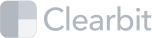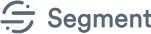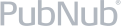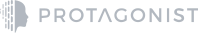# Top Machine Learning Algorithms Explained: How Do They Work?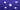Machine learning (ML) is a subfield of artificial intelligence (AI) that allows computers to learn to perform tasks and improve performance over time without being explicitly programmed. There are a number of important algorithms that help machines compare data, find patterns, or learn by trial and error to eventually calculate accurate predictions with no human intervention.

In many situations, machine learning tools can perform more accurately and much faster than humans. Uses range from driverless cars, to smart speakers, to video games, to data analysis, and beyond.

Let’s dive into different kinds of machine learning and the most-used algorithms to get an idea of how machine learning works.

## Types of Machine Learning Algorithms

To understand how machine learning algorithms work, we’ll start with the four main categories or styles of machine learning.

## Supervised vs Unsupervised Learning

A supervised learning model is fed sorted training datasets that algorithms learn from and are used to rate their accuracy. An unsupervised learning model is given only unlabeled data and must find patterns and structures on its own.

### Supervised Learning

Supervised learning is the most common approach to machine learning. These algorithms predict outcomes based on previously characterized input data. They’re “supervised” because models need to be given manually tagged or sorted training data that they can learn from.

A good example of this is a spam filter. Based on patterns in previously seen spam emails, like irregular text patterns, misspelled names, etc., supervised programs predict whether an email is “spam” or “not spam.” At the outset of spam detection, email applications weren’t entirely accurate. However, the more they have been trained, the more accurately they’ve been able to predict, to the point that they now rarely predict incorrectly.

Depending on the type of output, there are two types of supervised learning: classification and regression.

#### Classification

In classification in machine learning, the output always belongs to a distinct, finite set of “classes” or categories. Classification algorithms can be trained to detect the type of animal in a photo, for example, to output as “dog,” “cat,” “fish,” etc. However, if not trained to detect beyond these three categories, they wouldn’t be able to detect other animals.

Sentiment analysis is a good example of classification in text analysis. Models are trained to classify text as Positive, Negative, or Neutral.

Try out this free sentiment analysis classifier:

### Results

TagConfidence
Negative99.9%

As the model has been thoroughly trained, it has no problem predicting the text with full confidence.

#### Regression

Regression, on the other hand, outputs for probability as a continuous number value between 0 and 1. It predicts quantities or the probability that something will occur, like property values in a particular location or the effects an economic crisis may have on the stock market.

The example below shows a probability between 0 and 1 that a given individual would pay back a bank loan:### Unsupervised Learning

When it comes to unsupervised machine learning, the data we input into the model isn’t presorted or tagged, and there is no guide to a desired output. Unsupervised learning is generally used to find unknown relationships or structures in training data. It can remove data redundancies or superfluous words in a text or uncover similarities to group datasets together.

Clustering algorithms are common in unsupervised learning and can be used to recommend news articles or online videos similar to ones you’ve previously viewed.

### Semi-Supervised Learning

Semi-supervised learning is just what it sounds like, a combination of supervised and unsupervised. It uses a small set of sorted or tagged training data and a large set of untagged data. The models are guided to perform a specific calculation or reach a desired result, but they must do more of the learning and data organization themselves, as they’ve only been given small sets of training data.

Even though they have been trained with fewer data samples, semi-supervised models can often provide more accurate results than fully supervised and unsupervised models. They combine the overfitting and underfitting of data, problems presented by supervised and unsupervised learning, to deliver an output that ‘generalizes well.’ In more simple terms, semi-supervised algorithms represent a middle ground of learning too much and not learning too much from a dataset. Semi-supervised is often a top choice for data analysis because it’s faster and easier to set up and can work on massive amounts of data with a small sample of labeled data.

### Reinforcement Learning

Reinforcement learning is explained most simply as “trial and error” learning. In reinforcement learning, a machine or computer program chooses the optimal path or next step in a process based on previously learned information. Machines learn with maximum reward reinforcement for correct choices and penalties for mistakes.

This can be seen in robotics when robots learn to navigate only after bumping into a wall here and there – there is a clear relationship between actions and results. Like unsupervised learning, reinforcement models don’t learn from labeled data. However, reinforcement models learn by trial and error, rather than patterns.

## Top Algorithms Used in Machine Learning

Machine learning is an expansive field and there are billions of algorithms to choose from. The one you use all depends on what kind of analysis you want to perform. And even then, there can be multiple ways to get there. The algorithms below, however, are some of the best and most powerful.

Top machine learning algorithms:

### Linear Regression

Linear regression predicts a Y value, given X features. Machine learning works to show the relationship between the two, then the relationships are placed on an X/Y axis, with a straight line running through them to predict future relationships.

In sentiment analysis, linear regression calculates how the X input (meaning words and phrases) relates to the Y output (opinion polarity – positive, negative, neutral). This will determine where the text falls on the scale of “very positive” to “very negative” and between.

### Logistic Regression

Logistic regression is an algorithm that predicts binary outcome, a positive or negative conclusion: Yes/No, Existence/Non-existence, Pass/Fail. It means, simply, something happens or does not.

Variables are calculated against each other to determine the 0/1 outcome (one of two categories):

P(Y=1|X) or P(Y=0|X)

The independent variables can be categorical or numeric, but the dependent variable is always categorical: the probability of dependent variable Y, given independent variable X

This can be used to determine the object in a photo or video image (cup, bowl, spoon, etc.) with each object given a probability between 0 and 1, or to calculate the probability of a word having a positive or negative connotation (0, 1, or on a scale between).

### Naive Bayes Classifier

When used in text analysis, Naive Bayes is a family of probabilistic algorithms that use Bayes’ Theorem to calculate the possibility of words or phrases falling into a set of predetermined “tags” (categories) or not. This can be used on news articles, customer reviews, emails, general documents, etc.

They calculate the probability of each tag for a given text, then output for the highest probability:The probability of A, if B is true, is equal to the probability of B, if A is true, times the probability of A being true, divided by the probability of B being true.

There can be multiple tags assigned to any given use case (problem), but they are each calculated individually. When tagging customer reviews, for example, we could use tags like Pricing, Usability, Features, etc., but each piece of text would be calculated against only one tag at a time:### Support Vector Machines

A support vector machine (SVM) is a supervised machine learning model used to solve two-group classification models. Unlike Naive Bayes, SVM models can calculate where a given piece of text should be classified among multiple categories, instead of just one at a time.

SVM allows for more in-depth results because it is multidimensional. In the below, we’ll use tags “red” and “blue,” with data features “X” and “Y.” The classifier is trained to place red or blue on the X/Y axis.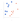The SVM then assigns a hyperplane that best separates the tags. In two dimensions this is simply a line (like in linear regression), with red on one side of the line and blue on the other.

This video tutorial explains how the optimal hyperplane is found – the best hyperplane is the one with the largest distance between each tag.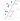However, some data is more complex and  it may not be possible to draw a single line to classify the data into two categories: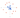Thanks to the “multi-dimensional” power of SVM, more complex data will actually produce more accurate results. Imagine the above in three dimensions, with a Z-axis added, so it becomes a circle.

Mapped back to two dimensions the optimal hyperplane would look like this: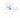### Decision Tree or CART (Classification and Regression Tree)

Decision tree, also known as classification and regression tree (CART), is a supervised learning algorithm that works great on text classification problems because it can show similarities and differences on a hyper minute level. It, essentially, acts like a flow chart, breaking data points into two categories at a time, from “trunk,” to “branches,” then “leaves,” where the data within each category is at its most similar.

This creates classifications within classifications, showing how the precise leaf categories are ultimately within a trunk and branch category.

### Random Forest

Random forest is an expansion of decision tree and useful because it fixes the decision tree’s dilemma of unnecessarily forcing data points into a somewhat improper category.

It works by first constructing decision trees with training data, then fitting new data within one of the trees as a “random forest.” Put simply, random forest averages your data to connect it to the nearest tree on the data scale.

### K-nearest Neighbors

K-nearest neighbors or “k-NN” is a pattern recognition algorithm that uses training datasets to find the k closest related members in future examples.

Used in text analysis, we would calculate to place a given word or phrase within the category of its nearest neighbor. K is decided by a plurality vote of its neighbors.  If k = 1, then it would be placed in the class nearest 1.

### K-Means

K-means is an iterative algorithm that uses clustering to partition data into non-overlapping subgroups, where each data point is unique to one group.

Once the algorithm identifies k clusters and has allocated every data point to the nearest cluster,  the geometric cluster center (or centroid) is initialized. First, the dataset is shuffled, then K data points are randomly selected for the centroids without replacement. Secondly, the algorithm iterates until the centroids no longer move. Or, in other words, the data points assigned to clusters remain the same.

### Apriori

Apriori calculates the probability that a data point will belong to a given dataset if one or more other data points are present. It’s a Boolean association algorithm using prior knowledge (a priori) of frequent or recurring dataset properties. If X, then Y is likely to occur: X→ Y.

Apriori calculations contain two steps:

1. Identify frequently related datasets that occur/relate to each other a predetermined minimum number of times.
2. Determine minimum association rules for new data points to relate to these datasets.

In text analysis, we can see a basic example of how this machine learning algorithm works in relation to classification. If one of our classification tags is Pricing, our model would quickly be trained to understand that the word “price” should be associated with that tag, as well as related words, like “expensive,” and “cost.” However, the more the model is trained it would be able to learn that certain words, which individually may not be associated with Pricing, when used together, should, in fact, be classified as Pricing. Like, “high ticket item”: X {high+ticket} → Y {Pricing}.

### Principal Component Analysis (PCA)

PCA is a basic but powerful type of “dimension reduction” algorithm within unsupervised learning. It works by reducing the number of variables within a calculation to place the highest variance in the data into a new coordinate system. These new axes become “principal components.”

The first principal component forms a line that best continues the trajectory of the data and the next is orthogonal to the first, the third component to the second, and so on. Thus, PCA is able to capture the variance from one component to its neighbor but components that are not directly succeeding each other are not necessarily directly related.

## Wrap Up

Machine learning is a deep and sophisticated field with complex mathematics, myriad specialties, and nearly endless applications. The algorithms and styles of learning above are just a dip of the toe into the vast ocean of artificial intelligence.

Machine learning algorithms are only continuing to gain ground in fields like finance, hospitality, retail, healthcare, and software (of course). They deliver data-driven insights, help automate processes and save time, and perform more accurately than humans ever could.

But you don’t have to hire an entire team of data scientists and coders to implement top machine learning tools into your business. No code SaaS text analysis tools like MonkeyLearn are fast and easy to implement and super user-friendly.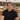#### TobiasGeisler Mesevage

August 28th, 2020

## Posts you might like...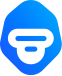## Text Analysis with Machine Learning

Turn tweets, emails, documents, webpages and more into actionable data. Automate business processes and save hours of manual data processing.